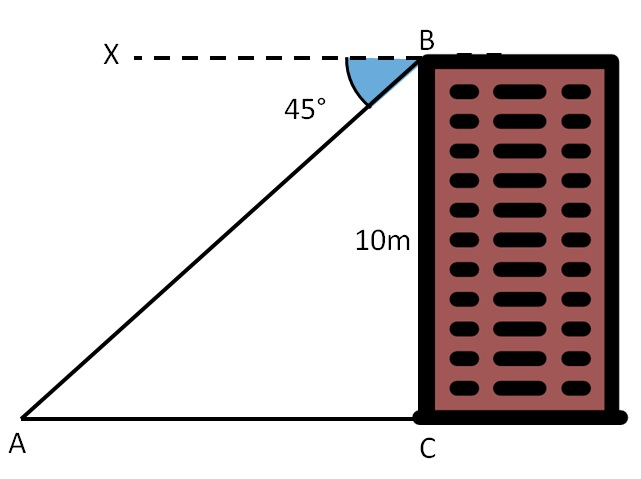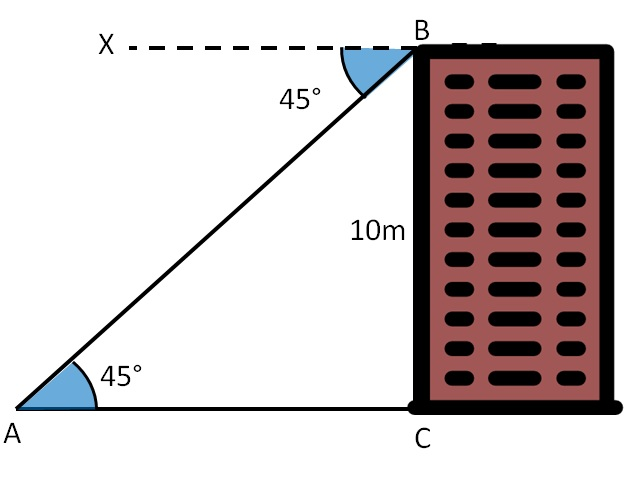Questions easy to difficult

Chapter 9 Class 10 Some Applications of Trigonometry
Concept wise
• Questions easy to difficult

Suppose angle of depression from top of the tower to point A is 45°. And height of tower is 10 m. What is the distance of point A from the building?Let building be BC

So, angle of depression from point B is 45°

and Height = BC = 10 m

BX is the horizontal

Now, we need to find the distance of point A from the building

Now, lines BX and AC are parallel,

and AB is the transversal

So, Alternate angles are equal

∴ ∠ BAC = ∠ XBA

∴ ∠ BAC = 45°Now, we need to find base AC

And we are given height 10 m.

So, we can use tan

As

tan A = Side opposite to angle A / Side adjacent to angle A

tan 45° = BC / AC

1 = 10/ AC

AC = 10 m

∴ Distance AC is 10 m

Learn in your speed, with individual attention - Teachoo Maths 1-on-1 Class